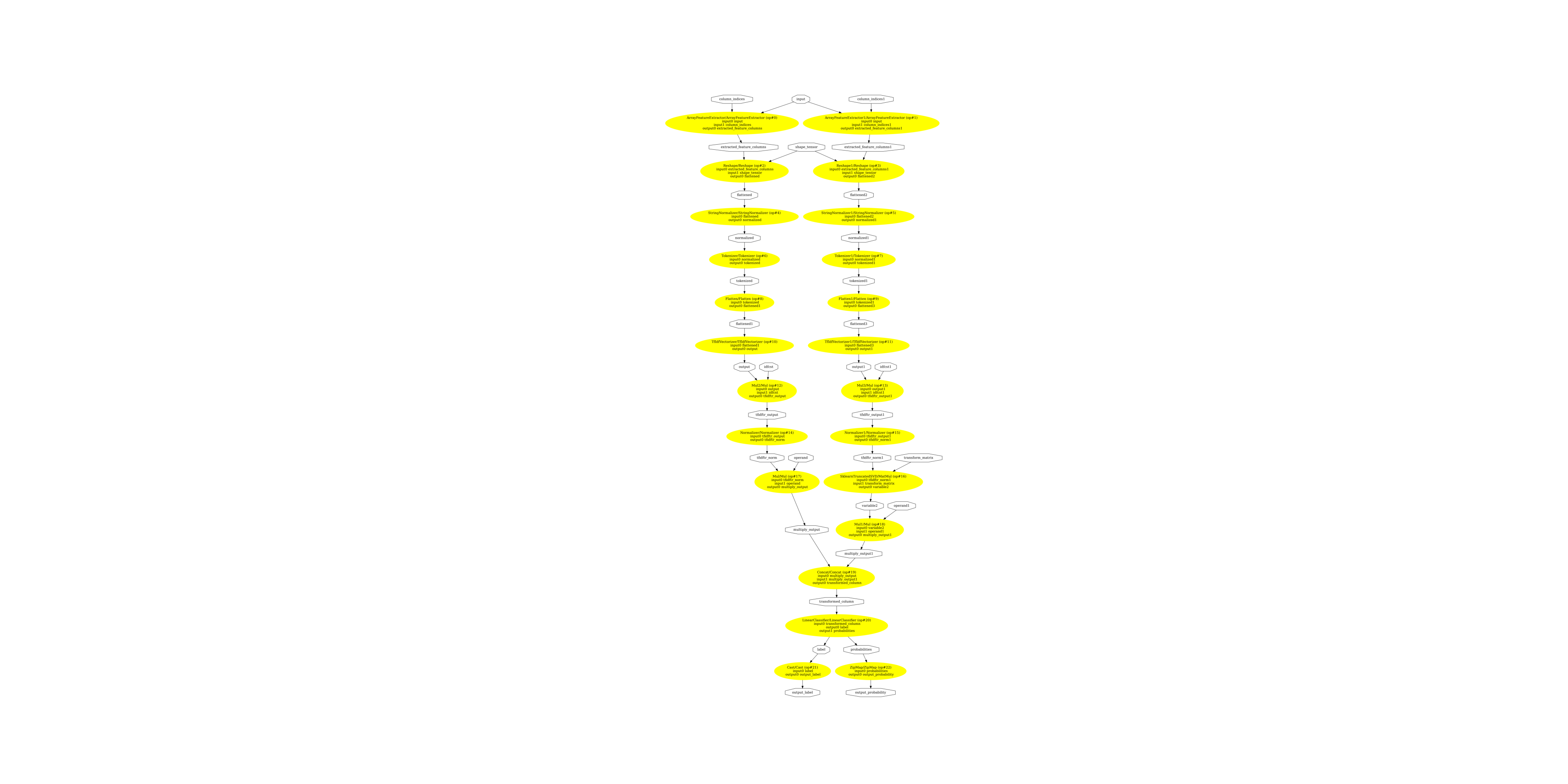# TfIdfVectorizer with ONNX#

This example is inspired from the following example: Column Transformer with Heterogeneous Data Sources which builds a pipeline to classify text.

## Train a pipeline with TfidfVectorizer#

It replicates the same pipeline taken from scikit-learn documentation but reduces it to the part ONNX actually supports without implementing a custom converter. Let’s get the data.

```import matplotlib.pyplot as plt
import os
from onnx.tools.net_drawer import GetPydotGraph, GetOpNodeProducer
import onnxruntime as rt
from skl2onnx.common.data_types import StringTensorType
from skl2onnx import convert_sklearn
import numpy as np

from sklearn.base import BaseEstimator, TransformerMixin
from sklearn.datasets import fetch_20newsgroups

try:
from sklearn.datasets._twenty_newsgroups import (
strip_newsgroup_footer,
strip_newsgroup_quoting,
)
except ImportError:
# scikit-learn < 0.24
from sklearn.datasets.twenty_newsgroups import (
strip_newsgroup_footer,
strip_newsgroup_quoting,
)
from sklearn.decomposition import TruncatedSVD
from sklearn.feature_extraction.text import TfidfVectorizer
from sklearn.pipeline import Pipeline
from sklearn.compose import ColumnTransformer
from sklearn.metrics import classification_report
from sklearn.linear_model import LogisticRegression

# limit the list of categories to make running this example faster.
categories = ["alt.atheism", "talk.religion.misc"]
train = fetch_20newsgroups(
random_state=1,
subset="train",
categories=categories,
)
test = fetch_20newsgroups(
random_state=1,
subset="test",
categories=categories,
)
```

The first transform extract two fields from the data. We take it out form the pipeline and assume the data is defined by two text columns.

```class SubjectBodyExtractor(BaseEstimator, TransformerMixin):
"""Extract the subject & body from a usenet post in a single pass.
Takes a sequence of strings and produces a dict of sequences. Keys are
`subject` and `body`.
"""

def fit(self, x, y=None):
return self

def transform(self, posts):
# construct object dtype array with two columns
# first column = 'subject' and second column = 'body'
features = np.empty(shape=(len(posts), 2), dtype=object)
for i, text in enumerate(posts):
bod = strip_newsgroup_footer(bod)
bod = strip_newsgroup_quoting(bod)
features[i, 1] = bod

prefix = "Subject:"
sub = ""
if line.startswith(prefix):
sub = line[len(prefix) :]
break
features[i, 0] = sub

return features

train_data = SubjectBodyExtractor().fit_transform(train.data)
test_data = SubjectBodyExtractor().fit_transform(test.data)
```

The pipeline is almost the same except we remove the custom features.

```pipeline = Pipeline(
[
(
"union",
ColumnTransformer(
[
("subject", TfidfVectorizer(min_df=50, max_features=500), 0),
(
"body_bow",
Pipeline(
[
("tfidf", TfidfVectorizer()),
("best", TruncatedSVD(n_components=50)),
]
),
1,
),
# Removed from the original example as
# it requires a custom converter.
# ('body_stats', Pipeline([
#   ('stats', TextStats()),  # returns a list of dicts
#   ('vect', DictVectorizer()),  # list of dicts -> feature matrix
# ]), 1),
],
transformer_weights={
"subject": 0.8,
"body_bow": 0.5,
# 'body_stats': 1.0,
},
),
),
# Use a LogisticRegression classifier on the combined features.
("logreg", LogisticRegression()),
]
)

pipeline.fit(train_data, train.target)
print(classification_report(pipeline.predict(test_data), test.target))
```
```              precision    recall  f1-score   support

0       0.69      0.78      0.73       285
1       0.75      0.66      0.70       285

accuracy                           0.72       570
macro avg       0.72      0.72      0.71       570
weighted avg       0.72      0.72      0.71       570
```

## ONNX conversion#

It is difficult to replicate the exact same tokenizer behaviour if the tokeniser comes from space, gensim or nltk. The default one used by scikit-learn uses regular expressions and is currently being implementing. The current implementation only considers a list of separators which can is defined in variable seps.

```seps = {
TfidfVectorizer: {
"separators": [
" ",
".",
"\\?",
",",
";",
":",
"!",
"\\(",
"\\)",
"\n",
'"',
"'",
"-",
"\\[",
"\\]",
"@",
]
}
}
model_onnx = convert_sklearn(
pipeline,
"tfidf",
initial_types=[("input", StringTensorType([None, 2]))],
options=seps,
target_opset=12,
)
```

And save.

```with open("pipeline_tfidf.onnx", "wb") as f:
f.write(model_onnx.SerializeToString())
```

Predictions with onnxruntime.

```sess = rt.InferenceSession("pipeline_tfidf.onnx", providers=["CPUExecutionProvider"])
print("---", train_data)
inputs = {"input": train_data[:1]}
pred_onx = sess.run(None, inputs)
print("predict", pred_onx)
print("predict_proba", pred_onx)
```
```--- [" Re: Jews can't hide from keith@cco."
'Deletions...\n\nSo, you consider the german poster\'s remark anti-semitic?  Perhaps you\nimply that anyone in Germany who doesn\'t agree with israely policy in a\nnazi?  Pray tell, how does it even qualify as "casual anti-semitism"? \nIf the term doesn\'t apply, why then bring it up?\n\nYour own bigotry is shining through.  \n-- ']
predict 
predict_proba [{0: 0.4396112561225891, 1: 0.5603887438774109}]
```

With scikit-learn:

```print(pipeline.predict(train_data[:1]))
print(pipeline.predict_proba(train_data[:1]))
```
```
[[0.72374074 0.27625926]]
```

There are discrepencies for this model because the tokenization is not exactly the same. This is a work in progress.

## Display the ONNX graph#

Finally, let’s see the graph converted with sklearn-onnx.

```pydot_graph = GetPydotGraph(
model_onnx.graph,
name=model_onnx.graph.name,
rankdir="TB",
node_producer=GetOpNodeProducer(
"docstring", color="yellow", fillcolor="yellow", style="filled"
),
)
pydot_graph.write_dot("pipeline_tfidf.dot")

os.system("dot -O -Gdpi=300 -Tpng pipeline_tfidf.dot")```(-0.5, 4939.5, 11475.5, -0.5)